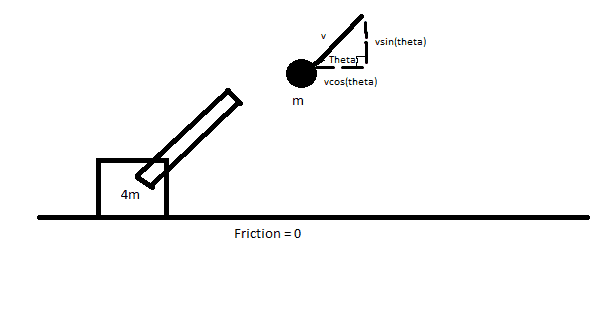# I need help with an AP Physics Momentum/Collision problem

AP Physics Momentum/Collision problem..

## Homework Statement"A projectile of mass M is fired at an angle Theta (with the horizontal) from a cannon of mass 4M and velocity V on a friction-less flat surface. Determine Theta such that the distance from the end of the cannon (the hole of the cannon) to the projectile's peak is at a maximum. Hint: Let v = 1."
Answer: Theta = 50.49 degrees."

Theta, Mass, and Velocity are variables.

## Homework Equations

change in Y distance = V(initial)T - 1/2gt^2
Not sure if there are any other ones..

## The Attempt at a Solution

I drew this out with the cannon, on a frictionless flat surface, firing a projectile as such. Then, from there, after drawing out the Velocity vector on the mass, I attempted to break up my velocity vectors into the proper dimensional component:

where the velocity in the X = vcos(theta)
and velocity in the Y = vsin(theta)

from there I have no clue what to do. I've tried a couple of different things but I'm not sure how my teacher obtained a numerical value as the answer. He said to let V = 1 which I am clueless when to apply that.

Thank you very much for helping!

Last edited:

## Answers and Replies

TSny
Homework Helper
Gold Member
Hello akitokunx. Welcome to PF!

It appears that you are to assume the projectile always comes out of the barrel with a fixed speed v relative to the ground no matter what angle the cannon is fired (despite the fact that the cannon will recoil at different speeds depending on the angle). I think that assumption will lead to the answer given.

Can you show some work on how to find the time for the projectile to reach max height? Can you express that time in terms of v, θ, and g? (You can access symbols like θ by clicking on the "Go Advanced" tab below the reply box.)

Can you express the horizontal recoil speed (u, say) of the cannon in terms of the initial speed v and θ of the projectile?

Umm for the horizontal recoil speed I used conservation of momentum:
Momentum Initial = Momentum Final

so 0 = mbullet*vbullet - mcannon*vcannon
vcannon = mbullet*vbullet/mcannon
mcannon = 4m, vbullet = vcos(θ), mbullet = m

so vcannon = mvcos(θ)/4m -> vcos(θ)/4

-----------------

For the height, I used
change in y = Vinitial(T) - 1/2gt^2

I worked it out to get t = (squarerootof 2)(change in Y)/g ... I'm not sure what I'm doing wrong here.

Thanks again for the help!

For every action there is an equal and opposite reaction.
From basic conservation of momentum (m1+m2)*vo=m1*v1+m2*v2
since vo=0, this equation yields vcannon=-1/4 vprojectile

Now then we can consider the fact that the cannon can only move in one dimension. So the effective velocity of the cannon is -1/4 vprojectile cosθ

From here find a function that defines the distance between the two as a function of time.
What I started with was a function relative to the origin, for each.
So Cannon distance to origin=(-1/4*vprojectile*cosθ)*t
Projectile distance= (vprojectile*cosθ*t)i+(vprojectile*sinθ*t+1/2*g*t2)j

From here, the distance between the two is √[(x2-x1)2+(y2-y1)2]

I will leave it up to you from here. Perhaps try to take a derivative and set it equal to 0 to find a maximum. Then solve for theta.

TSny
Homework Helper
Gold Member
so vcannon = mvcos(θ)/4m -> vcos(θ)/4
Good. The horizontal component of momentum is conserved. So, now you have a good expression for the recoil speed of the cannon.
For the height, I used
change in y = Vinitial(T) - 1/2gt^2
What is Vinitial here? Is it the initial speed of the projectile, v? Or is it only the y-component of the initial speed v? How would you express the y-component of the initial speed in terms of v and θ?
I worked it out to get t = (squarerootof 2)(change in Y)/g ... I'm not sure what I'm doing wrong here.
This is not correct. Looks like you somehow dropped the term involving Vinitial. Anyway, solving the y equation for t is not going to help.

The problem wants you to consider the time when the projectile is at its maximum height. So you need to find an expression for the time when the projectile is at max height. Think about what must be true at maximum height of a projectile and use that to find an expression for t.

Once you have t at max height you should be able to work out expressions for where the cannon is at that time as well as the x and y positions of the projectile at that time. These expressions will be in terms of v and θ.